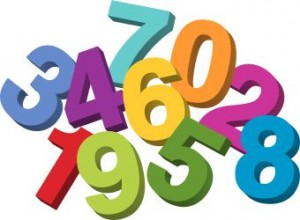# Random Seed: Definition

Statistics Definitions > Random Seed

## What is a Random Seed?A random seed is a starting point in generating random numbers.A random seed specifies the start point when a computer generates a random number sequence. This can be any number, but it usually comes from seconds on a computer system’s clock (Henkemans & Lee, 2001).

A computer counts seconds from January 1, 1970 — a system called Unix time. At time I am writing this sentence it is 11:01:46 EST on February 5, 2017: 1486310506 seconds after 1-1-1970 (you can find the current Unix time here). Having such a large range for seeds (0 to 1+ billion) pretty much ensures that you don’t get the same random seed isn’t used twice — unless you want to.

For example, let’s say you wanted to generate a random number in Excel (Note: Excel sets a limit of 9999 for the seed). If you enter a number into the Random Seed box during the process, you’ll be able to use the same set of random numbers again. If you typed “77” into the box, and typed “77” the next time you run the random number generator, Excel will display that same set of random numbers. If you type “99”, you’ll get an entirely different set of numbers. But if you revert back to a seed of 77, then you’ll get the same set of random numbers you started with.

## Why are Seeds Needed?

Computers don’t generate truly random numbers—they are deterministic, which means that they operate by a set of rules. You can mimic randomness by specifying a set of rules. For example, “take a number x, add 900 +x, then subtract 52.” In order for the process to start, you have to specify a starting number, x (the seed). Let’s take the starting number 77:

• Add 900 + 77 = 977
• Subtract 52 = 925

Following the same algorithm, the second “random” number would be:

• 900 + 925 = 1825
• Subtract 52 = 1773

This simple example follows a pattern, but the algorithms behind computer number generation are much more complicated (based on distributions like the Bernoulli distribution or Poisson distribution), mimicking randomness much better than I can do here. But the process still follows a pattern, which will be repeated the next time you enter 77 or 99, or whatever number you choose into the “random seed” box.

How to Use the Random Number Generator in Excel

Watch the video or read the steps below:You have two random number generator options in Excel: the RAND function (a variation is the RANDBETWEEN function) and the Data Analysis Toolpak.

### Random Number Generator: The RAND Function

Step 1: Type “=RAND()” into an empty cell.
Step 2: Press “ENTER.” This generates a random number between 0 and 1.
Step 3: Click the blue box at the bottom right of the cell to drag the formula up a column or across a row. This generates more random numbers.

### Random Number Generator in Excel: The RANDBETWEEN Function

Step 1: Type “=RANDBETWEEN(a,b)” into an empty cell, where a,b is the range you want to pick numbers from. For example, =RANDBETWEEN(1,100) will generate a random number between 1 and 100.
Step 2: Press “ENTER.” This generates a random number between the number you specified.
Step 3: Click the blue box at the bottom right of the cell to drag the formula up a column or across a row. This generates more random numbers.

### Data Analysis Toolpak

Step 1: Click the “Data” tab and then click “Data Analysis.” If you don’t see Data Analysis, load the Data Analysis Toolpak.
Step 2: Type how many columns you want to populate in the Number of Variables Box. For example, you might want to display 20 random numbers in 10 columns (2 random numbers per column); Type “10” into the box.
Step 3: Type how many random numbers you want in each column into the Number of Random Numbers box. In this example (2 numbers in 10 columns), type “2” into the box.
Step 4: Select a distribution. For example, select “Normal Distribution.”
Step 5: Type the remaining variables into the boxes. For example, for Normal Distribution type in the mean and standard deviation.
Step 6: Tell Excel where you want the output. For example, click the radio button next to “New Worksheet.”
Step 7: Press “OK.”

Tip: Enter a number into the Random Seed box if you anticipate needing the exact same set of numbers again. For example, type “99” into the box before pressing “OK.” The next time you run the random number generator, type 99 into the Random Seed box and Excel will display that same set of random numbers.

Check out our YouTube channel for more Excel/stats help and tips!

References:
Henkemans, D & Lee, M. (2001). C++ Programming for the Absolute Beginner. Cengage Learning.

CITE THIS AS:
Stephanie Glen. "Random Seed: Definition" From StatisticsHowTo.com: Elementary Statistics for the rest of us! https://www.statisticshowto.com/random-seed-definition/